# Analysis with Raster Data Using R and rasterList Package: saving any kind of data in a grid cell

## Abstract

The rasterList package has been developed to create a S4 class object that can manage complex operations and objects in a spatially gridded map, i.e. a raster map. The rasterList-Class (Cordano and Others (2018)) object differs from a traditional raster map by the fact that each cell can contain a generic object instead of numeric values on one or more bands or layers. The raster of objects,i.e. the RasterList-Class object, consists on a rasterLayer-Class (Hijmans (2016)) connected to a generic list: one list element for each raster cells. It allows to write few lines of R code for complex map algebra. Furthermore, in accordance with the existing classes defined in the R environment, each cell of the raster is “occupied” by an an istanced object. This may be of big utility in most applications, e.g. environmental modeling.

## Introduction

Nowadays the availability of spatially gridded coverages , often obtained by distributed biophysical/environmental models or remote sensed observations (e. g. CHIRPS rainfall (Funk et al. (2015))), leads to the fact that normal operations on time series analysis and statistics must be replicated in each pixel or cell of the spatially gridded domain (raster). Based on raster package (Hijmans (2016)), a S4 class has been created such that results of complex operations or speficfic R (e.g, S3 or S4) objects can be executed on each cells of a raster map. The raster of objects contains the traditional raster map with the addition of a list of generic objects: one object for each raster cells. In such a way, different kinds of objects, e.g. the probability distribution of the mapped random variable or an htest, lm ,glm or function class objects (Chambers (1992),Hastie and Pregibon (1992)), can be saved for each cell, in order that the user can save intermediate results without loosing any geographic information. This new object class is implemented in a new package RasterList. Two applications are presented:

• Creation of a 2D/3D modeled map of soil water retention curve based on existing soil properties map and state-of-art empirical formulation (e.g. VanGenuchten (1980));
• Processing spatial gridded datasets of daily or monthly precipitation (e. g. Funk et al. (2015)) for a trend or frequancy analysis (estimation of the return period of critical events).

## Application 1

The application 1 is the estimation of the mathematical relationships between soil water content $$\theta$$ and soil water pressure $$\psi$$, i.e. soil water retention curve. Soil water retention curve is widely used in subsurface water/groundwater hydrological modeling because it allows to close the water balance equation. Generally some theoretical formulas are used to model this curve in function of soil properties. In this examples, given a raster map of soil properties, a map of soil water retention curves is produced. First of all, in absence of detailed soil information (see also the use in Kollet et al. (2017)), soil water curve is defined with the following empirical formula (VanGenuchten (1980)):

$\theta = \theta_{res}+(\theta_{sat}-\theta_{res})*(1+ |\alpha \psi|^n)^{-m} \qquad \psi \leq 0$ $\theta = \theta_{sat} \qquad \psi>0$

where $$\theta$$ is the volumetric water content (e.g. water volume per soil volume) which ranges between residual water content $$\theta_{res}$$ and saturated water content $$\theta_{sat}$$. $$\alpha$$,$$n$$ and $$m$$ are parameters assuming to be depend on soil type. An estimation of the parameter $$\theta_{sat}$$,$$\theta_{res}$$,$$\alpha$$,$$m$$,$$n$$ value in function of soil type is given through the following table (Maidment (1993)):

library(soilwater)
library(stringr)
soilparcsv <- system.file("external/soil_data.csv",package="soilwater")
knitr::kable(soilpar,caption="Average value of Van Genuchten's parameter per each soil type")
Average value of Van Genuchten’s parameter per each soil type
type swc rwc alpha n m Ks_m_per_hour Ks_m_per_sec
sand 0.44 0.02 13.8 2.09 0.52153 0.15000 4.17e-05
loamy sand 0.44 0.04 11.5 1.87 0.46524 0.10000 2.78e-05
sandy loam 0.45 0.05 6.8 1.62 0.38272 0.03900 1.08e-05
loam 0.51 0.04 9.0 1.42 0.29577 0.03300 9.20e-06
silt loam 0.50 0.03 4.8 1.36 0.26471 0.00900 2.50e-06
sandy clay loam 0.40 0.07 3.6 1.56 0.35897 0.00440 1.20e-06
clay loam 0.46 0.09 3.9 1.41 0.29078 0.00480 1.30e-06
silty clay loam 0.46 0.06 3.1 1.32 0.24242 0.00130 4.00e-07
sandy clay 0.43 0.11 3.4 1.40 0.28571 0.00073 2.00e-07
silty clay 0.48 0.07 2.9 1.26 0.20635 0.00076 2.00e-07
clay 0.48 0.10 2.7 1.29 0.22481 0.00041 1.00e-07
soilpar$color <- str_sub(rainbow(nrow(soilpar)),end=7) ## Only first 7 characters of HTML code is considered. It it is assumed that each cell of a raster maps has its own soil water retention curve. Therefore to map the soil water retention curve and not only its parameters, RasterList package is loaded: library(rasterList) #> Loading required package: raster #> Loading required package: sp The study area is is the area of the ‘meuse’ dataset’ ( (n.d.)): library(sp) library(sf) #> Linking to GEOS 3.10.2, GDAL 3.4.3, PROJ 8.2.0; sf_use_s2() is TRUE data(meuse) ## USE sf help(meuse) data(meuse.grid) help(meuse.grid) A soil map is available from soil type according to the 1:50 000 soil map of the Netherlands. In the Meuse site, the following soil type are present • 1 = Rd10A (Calcareous weakly-developed meadow soils, light sandy clay); • 2 = Rd90C/VII (Non-calcareous weakly-developed meadow soils, heavy sandy clay to light clay); • 3 = Bkd26/VII (Red Brick soil, fine-sandy, silty light clay) which can be respectively assimilated as: soiltype_id <- c(1,2,3) soiltype_name <- c("sandy clay","clay","silty clay loam") ## Then soilpar_s <- soilpar[soilpar$type %in% soiltype_name,]
is <- order(soilpar_s[,1],by=soiltype_name)
soilpar_s <- soilpar_s[is,]
soilpar_s$id <- soiltype_id A geographic view of Meuse test case is available though leaflet package (Cheng, Karambelkar, and Xie (2018)): library(rasterList) library(soilwater) library(leaflet) set.seed(1234) data(meuse.grid) data(meuse) coordinates(meuse.grid) <- ~x+y coordinates(meuse) <- ~x+y gridded(meuse.grid) <- TRUE ### +init=epsg:28992 proj4string(meuse) <- CRS("+init=epsg:28992") proj4string(meuse.grid) <- CRS("+init=epsg:28992") ## Not run: meuse <- as(meuse,"sf") soilmap <- as.factor(stack(meuse.grid)[['soil']]) ###elevmap <- rasterize(x=meuse,y=soilmap,field="elev",fun=mean) ref_url <- "https://{s}.tile.opentopomap.org/{z}/{x}/{y}.png" ref_attr <- 'Map data: &copy; <a href="http://www.openstreetmap.org/copyright">OpenStreetMap</a>, <a href="http://viewfinderpanoramas.org">SRTM</a> | Map style: &copy; <a href="https://opentopomap.org">OpenTopoMap</a> (<a href="https://creativecommons.org/licenses/by-sa/3.0/">CC-BY-SA</a>)' opacity <- 0.6 color <- colorFactor(soilpar_s$color,level=soilpar_s$id) labFormat <- function(x,values=soilpar_s$type){values[as.integer(x)]}

leaf2 <- leaf1 %>% addRasterImage(soilmap,opacity=opacity,color=color) %>% ##addLegend(position="bottomright",pal=color,values=soilpar_s$id,labels=soilpar_s$type,title="Soil Type",labFormat=labFormat)
addLegend(position="bottomright",colors=soilpar_s$color,labels=soilpar_s$type,title="Soil Type",opacity=opacity)

leaf2

Therefore, a map of each VanGenuchten (1980)’s formula parameter can be obtained as follows (The names of saturated and residual water contents swc and rwc mean $$\theta_{sat}$$ and $$theta_{res}$$ according to the the arguments of swc function of soilwater package (Cordano, Andreis, and Zottele (2017)))


soil_parameters_f <- function (soiltype,sp=soilpar_s) {
o <- sp[soiltype,c("swc","rwc","alpha","n","m")]
names(o) <- c("theta_sat","theta_res","alpha","n","m")
return(o)
}
soil_parameters_rl <- rasterList(soilmap,FUN=soil_parameters_f)

A RasterList-class object has been created and each cell contains a vector of soil water parameters:

soil_parameters <- stack(soil_parameters_rl)
soil_parameters
#> class      : RasterStack
#> dimensions : 104, 78, 8112, 5  (nrow, ncol, ncell, nlayers)
#> resolution : 40, 40  (x, y)
#> extent     : 178440, 181560, 329600, 333760  (xmin, xmax, ymin, ymax)
#> crs        : +proj=sterea +lat_0=52.1561605555556 +lon_0=5.38763888888889 +k=0.9999079 +x_0=155000 +y_0=463000 +ellps=bessel +units=m +no_defs
#> names      : theta_sat, theta_res,   alpha,       n,       m
#> min values :   0.43000,   0.06000, 2.70000, 1.29000, 0.22481
#> max values :   0.48000,   0.11000, 3.40000, 1.40000, 0.28571

A map of saturated water content (porosity) can be visualized as follows:

theta_sat <- soil_parameters[["theta_sat"]]
color <- colorNumeric("Greens",domain=theta_sat[])

leaf3 <- leaf1 %>% addRasterImage(theta_sat,color=color,opacity=opacity) %>%
addLegend(position="bottomright",pal=color,values=theta_sat[],opacity=opacity,title="Porosity")

Let consider 3 points of interest in the Meuse located (latitude and logitude coordinates) as follows:

lat <- 50.961532+c(0,0,0.02)
lon <-  5.724514+c(0,0.01,0.0323)
name <- c("A","B","C")

points <- data.frame(lon=lon,lat=lat,name=name)
print(points)
#>        lon      lat name
#> 1 5.724514 50.96153    A
#> 2 5.734514 50.96153    B
#> 3 5.756814 50.98153    C
coordinates(points) <- ~lon+lat
proj4string(points) <- CRS("+proj=longlat  +ellps=WGS84")
points <- as(points,"sf")

leaf3 %>% addMarkers(lng=st_coordinates(points)[,"X"],lat=st_coordinates(points)[,"Y"],label=points$name) They are indexed as follows in the raster map:  #####points$icell <- cellFromXY(soil_parameters,spTransform(points,projection(soil_parameters))) ##
points$icell <- cellFromXY(soil_parameters,as_Spatial(st_transform(points,crs=projection(soil_parameters)))) where icell is a vector of integer numbers corresponding to the cells of the analyzed raster map contained the points A,B and C. Information on these points can be extracted as follows: soil_parameters[points$icell]
#>      theta_sat theta_res alpha    n       m
#> [1,]      0.48      0.10   2.7 1.29 0.22481
#> [2,]      0.48      0.10   2.7 1.29 0.22481
#> [3,]      0.43      0.11   3.4 1.40 0.28571

Using swc , a function that returns a soil water function from soil parameters is defined as follows:

library(soilwater)

swc_func <- function(x,...) {
o <- function(psi,y=x,...) {

args <- c(list(psi,...),as.list(y))
oo <- do.call(args=args,what=get("swc"))
}
return(o)
}

And in case that it is applied to point A (i.e. setting the soil parameters of point A), it is:

soilparA <- soil_parameters[points$icell][1,] swcA <- swc_func(soilparA) where scwA is a function corresponsig to the Soil Water Retention Curve in point A that can be plotted as follows: library(ggplot2) psi <- seq(from=-5,to=1,by=0.25) title <- "Soil Water Retention Curve at Point A" xlab <- "Soil Water Pressure Head [m]" ylab <- "Soil Water Content (ranging 0 to 1) " gswA <- ggplot()+geom_line(aes(x=psi,y=swcA(psi)))+theme_bw() gswA <- gswA+ggtitle(title)+xlab(xlab)+ylab(ylab) gswA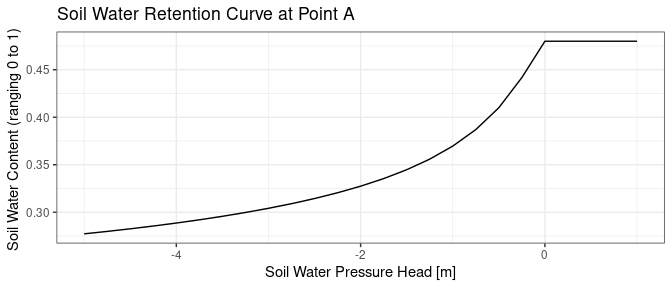Next steps, it is a generalization for the estimation for Soil Water Retention Curve in all cells of the raster map. This means to save the soil water retention curves as an R object for all the cells, and is possible though rasterList function: swc_rl <- rasterList(soil_parameters,FUN=swc_func) The rasterList-class object contains each soil water retention curve per each cell. Since the list element refered to each raster cell are function class, swc_rl is coerced to a single function defined all raster extension though rasterListFun swc_rl : swc_rlf <- rasterListFun(swc_rl) In such a way, the function swc_rlf can be used to estimate to estimate soil water function given a spatially unimorm value of soi water pressure head $$\psi$$ on all the region of interest. psi <- c(0,-0.5,-1,-2) names(psi) <- sprintf("psi= %1.1f m",psi) soil_water_content <- stack(swc_rlf(psi)) names(soil_water_content) <- names(psi) plot(soil_water_content,main=names(psi))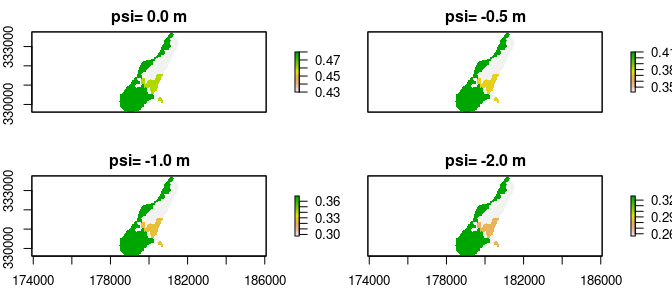Finally, assuming that $$\psi$$ may vary with space ranging between -0.5 m and -1.5 m with a spatial paraboloid-like profile centered in point A, a map for $$\psi$$ is calculated as follows: region <- raster(swc_rlf(0)) mask <- !is.na(region) region[] <- NA region[points$icell] <- 1
dist <- distance(region)

mdist <- max(dist[],na.rm=TRUE)

psi_max <- 0
psi_min <- -2
psi <- psi_max+(psi_min-psi_max)*(1-exp(-dist/mdist))
plot(psi)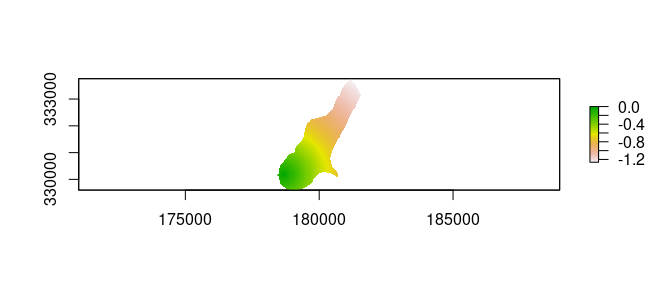color <- colorNumeric("Blues",domain=psi[])

leaf_psi <- leaf1 %>% addRasterImage(psi,color=color,opacity=opacity) %>%
addLegend(position="bottomright",pal=color,values=psi[],opacity=opacity,title="Psi") %>% addMarkers(lng=st_coordinates(points)[,"X"],lat=st_coordinates(points)[,"Y"],label=points$name) ##addMarkers(lng=points$lon,lat=points$lat,label=points$name)

leaf_psi

The corresponding map of $$\theta$$ is the following:

theta <- raster(swc_rlf(psi))
plot(theta)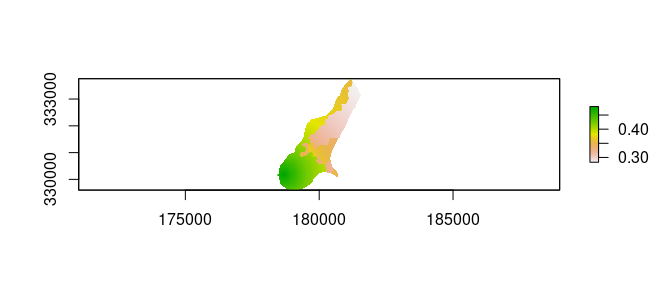color <- colorNumeric("Blues",domain=theta[])

leaf_psi <- leaf1 %>% addRasterImage(theta,color=color,opacity=opacity) %>%
addLegend(position="bottomright",pal=color,values=theta[],opacity=opacity,title="Psi") %>% addMarkers(lng=st_coordinates(points)[,"X"],lat=st_coordinates(points)[,"Y"],label=points$name) ##addMarkers(lng=points$lon,lat=points$lat,label=points$name)

leaf_psi

## Application 2

This application consists on an a time series analysis for each cell of a raster map. The application spatio-temporal gridded set of daily rainfall. The region of interest is an area covering the Cajamarca and Amozonas regions and its surroundings, Peru. This region is located in the northern part of the country and shares a border with Ecuador. Most of the territory covers the Andes Mountain Range and has heights around 2700 meters above sea level. The area has a subtropical highland climate (Cwb, in the Köppen climate classification) which is characteristic of high elevations at tropical latitudes. The region presents a semi-dry, temperate, semi-cold climate with presence of rainfall mostly on spring and summer (from October to March) with little or no rainfall the rest of the year. The western part of the study region is includes the Amazon Rainforest, one of the richest area in the world of biodiversity and water. This area is more rainy during all year than the part in the highlands. Anyway, the climate of the area is sensitive to ENSO oscillations. Anyway, the aim of this application is the creation of a work flow that can be applied to other areas of the world. The object of this session is a trend and raturn-period analysis of yearly aggregated precipitation starting from spatio-temporal gridded datasets available.
As input data, monthly precipitation rasters taken from the Climate Hazards Group InfraRed Precipitation with Station dataset (CHIRPS) (Funk et al. (2015)) have been used. CHIRPS is is a 30+ year quasi-global rainfall dataset. The spatial coverage spans all longitudes between the latitudes -50 degrees (Southern Hemisphere) and 50 degrees (Northern Hemisphere), starting in 1981 to near-present. CHIRPS incorporates 0.05 degree resolution satellite imagery with in-situ station data to create gridded rainfall time series for trend analysis and seasonal drought monitoring.

library(rasterList)
##precf <- system.file("map/Mekrou_precipitation.grd", package="rasterList")
#precf <- system.file("map/cajamarca_monthly_precipitation.grd", package="rasterList")
##
##precf0 <- '/home/ecor/local/rpackages/jrc/rasterList_doc/map/cajamarca_monthly_precipitation_vL.grd'
##precf <- '/home/ecor/local/rpackages/jrc/rasterList/inst/map/cajamarca_monthly_precipitation_vL2.grd'

##prec0 <- stack(precf0)
##prec <- aggregate(prec0,fact=2,na.rm=TRUE,fun=mean,filename=precf,overwrite=TRUE)
####
precf <- system.file("map/cajamarca_monthly_precipitation_vL2.grd", package="rasterList")
prec <- stack(precf)

Total annual precipitation can be computed through an aggregation of monthly data with a sum function aggregation and lubridate package (Grolemund and Wickham (2011)).


###  independent and identically distributed (i.i.d.
library(lubridate)

dates <- as.Date(names(prec),format="X%Y.%m.%d")
names(dates) <- names(prec)

years <- year(dates)
months <- month(dates)

im <- which(months<8)
years[im] <- years[im]-1

The meteorological year used for aggregation start on 1st August, due to the fact that the region of interest is located in Southern Hemishere. Because precipitation dataset is not aligned with this definitions of the year, it is checked that all meteorological years shall contain 12 months:


lseason <- tapply(X=dates,FUN=length,INDEX=years)
years_c <- names(lseason)[which(lseason==12)]
ic <- which(years %in% years_c)
prec <- prec[[ic]]
years <- years[ic]
months <- months[ic]

Then, it is:

  ###
prec_sum <- stackApply(prec,fun=sum,indices=years-years+1)
names(prec_sum) <- years+as.numeric(str_replace_all(names(prec_sum),"index_",""))-1
prec_sum
#> class      : RasterBrick
#> dimensions : 32, 17, 544, 37  (nrow, ncol, ncell, nlayers)
#> resolution : 0.1, 0.1  (x, y)
#> extent     : -79.45, -77.75, -7.8, -4.6  (xmin, xmax, ymin, ymax)
#> crs        : +proj=longlat +datum=WGS84 +no_defs
#> source     : memory
#> names      :    X1981,    X1982,    X1983,    X1984,    X1985,    X1986,    X1987,    X1988,    X1989,    X1990,    X1991,    X1992,    X1993,    X1994,    X1995, ...
#> min values : 24.88043, 33.30489, 27.41310, 21.51621, 21.12703, 25.37087, 24.40319, 22.74562, 22.79456, 22.32688, 24.08060, 28.48039, 23.44029, 21.85395, 22.47454, ...
#> max values : 3521.122, 3370.616, 3895.696, 3326.045, 3169.820, 3046.307, 2366.974, 2895.696, 2745.102, 2937.825, 2310.628, 2854.240, 3189.027, 2719.208, 2964.168, ...

A quick view of the total annual precipitation in the region is proposed below.



library(leaflet)

ref_url <- "https://server.arcgisonline.com/ArcGIS/rest/services/World_Topo_Map/MapServer/tile/{z}/{y}/{x}"
ref_attr <- "Tiles &copy; Esri &mdash; Esri, DeLorme, NAVTEQ, TomTom, Intermap, iPC, USGS, FAO, NPS, NRCAN, GeoBase, Kadaster NL, Ordnance Survey, Esri Japan, METI, Esri China (Hong Kong), and the GIS User Community"

opacity <- 0.6
r <- mean(prec_sum) ###prec_slm[["l_1"]]
#### Domain
mm <- range(r[])
buffer <- 200
mm <- mm+c(-1,1)*buffer
minzero <- TRUE
if (minzero==TRUE) mm <- 0 ## Precipitation cannot be negative
step <- buffer ###10^(ceiling(log(mm)/log(10))-2)
domain=seq(from=mm,to=mm+step,by=step)

bins <- (length(domain))/2+1
color <- colorBin("YlGnBu", domain = domain,bin=bins)
values <- domain[domain<=max(r[])]

leaf_l_1

Mann-Kendall trend analysis (Pohlert (2018)) is performed in order to detect if annual precipitation is increasing or decreasing with time. Firstly the attention is focused on two check points in the region of interest going from the Andes higlands to the Amazon Rainforest: Cajamarca city and Imaza (Amozanas) (Angoretos and Iquitos - Loreto, Peru - are also reported in the data frame below but ignored and out of the study map):


pts <- data.frame(lat=c(-7.140278,-5.16,-1.55481,-3.784722),lon=c(-78.488889,-78.288889,-74.609001,-73.308333),name=c("Cajamarca","Imaza","Angoteros","Iquitos"),label=c("CJA","IMZ","ANG","IQT"),stringsAsFactors = FALSE)
pts <- pts[1:2,] ## only Cajamarca and Imaza
pts$icell <- cellFromXY(prec_sum,pts[c("lon","lat")]) head(pts) #> lat lon name label icell #> 1 -7.140278 -78.48889 Cajamarca CJA 435 #> 2 -5.160000 -78.28889 Imaza IMZ 97 leaf_l_1 %>% addMarkers(lng=pts$lon,lat=pts$lat,label=sprintf("%10.2f mm at %s",r[pts$icell], pts$label)) Then, Sen’slope is an estimation of the trend which can be computed through the function sens.slope function of trend package, the computation is extended to the all map using rasterList function: library(trend) sens.slope_ <- function(x,...) { condNA <- all(is.na(x)) if (condNA) x[] <- -9999 o <- sens.slope(x,...) if (condNA) o[] <- NA return(o) } prec_sum_sens_slope <- rasterList(prec_sum,FUN=sens.slope_) Then a trend analysis on total annual precipitation has been done. The sens_slope variable returns the Mann-Kandall correlation test and Sen’s slope for all raster cells. Focusing on check points, the test results for them are:  lsl <- as.list(prec_sum_sens_slope@list[pts$icell])
names(lsl) <- pts$label lsl #>$CJA
#>
#>  Sen's slope
#>
#> data:  x
#> z = 1.0594, n = 37, p-value = 0.2894
#> alternative hypothesis: true z is not equal to 0
#> 95 percent confidence interval:
#>  -2.546925  7.575050
#> sample estimates:
#> Sen's slope
#>    2.789173
#>
#>
#> $IMZ #> #> Sen's slope #> #> data: x #> z = 1.9488, n = 37, p-value = 0.05132 #> alternative hypothesis: true z is not equal to 0 #> 95 percent confidence interval: #> -0.3237387 19.1070198 #> sample estimates: #> Sen's slope #> 10.07568 From test results (null hypothesis: zero trend with time) , in Cajamarca (CJA) annual precipitation has no significant non-zero trend (p-value is greater than 0.05) whereas a trend versus time looks insigicant and can be accepted only if increasing the tests significance (p-value is greater than 0.1) in Imaza (IMZ). A graphical representation of mean annual precipitation and a web map of precipitation trend in all area are available below:  library(gridExtra) library(ggplot2) getTrend <- function(x,signif=0.05) { pval <- x$p.value
o <- x$estimates o[pval>signif] <- 0 return(o) } plotTrend <- function(x=prec_sum[pts$icell][1,],trend=NULL,time=1:length(x),signif=0.05,variable.name="VARNAME",title="Title",...) {
if (is.null(trend)) trend <- sens.slope_(x,...)
trend <- getTrend(x=trend,signif=signif)
print(trend)
xm <- mean(x)
ylab <- sprintf("%s an. [mm] from mean",variable.name)
mean <- sprintf("(mean: %5.2f mm)",xm)
o <- ggplot()+geom_col(aes(x=time,y=x-xm))+theme_bw()
o <- o+xlab("Time [years]")+ylab(ylab)
o <- o+ggtitle(paste0(title,"  ",mean))
intercept <- -trend*time
o <- o+geom_abline(mapping=NULL,intercept=intercept,slope=trend)
o <- o+theme(title =element_text(size=8))
return(o)

}

time <- as.numeric(str_replace(names(prec_sum),"X",""))

prec_sum_sens_slope_trend <- raster(prec_sum_sens_slope,FUN=getTrend)

ptstrend <- list()
for (i in 1:nrow(pts)){
ptstrend[[i]] <- plotTrend(prec_sum[pts$icell][i,],variable.name="MAP",title=sprintf("%s (%s)",pts$name[i],pts$label[i]),time=time) } #> Sen's slope #> 0 #> Sen's slope #> 0 do.call(what=grid.arrange,args=ptstrend)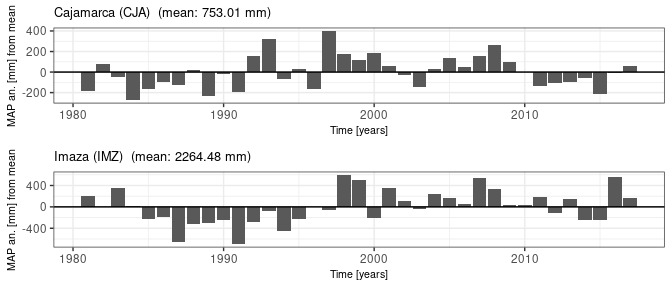r <- prec_sum_sens_slope_trend #### Domain mm <- range(r[],na.rm=TRUE) buffer <- 1 #0.01 mm <- mm+c(-1,1)*buffer minzero <- TRUE if (minzero==TRUE) mm <- 0 ## Precipitation cannot be negative step <- buffer ###10^(ceiling(log(mm)/log(10))-2) domain=seq(from=mm,to=mm+step,by=step) bins <- (length(domain))/2+1 color <- colorBin("YlGnBu", domain =domain,bin=bins) values <- domain[domain<=max(r[])] leaf_trend <- leaf %>% addRasterImage(r,opacity=opacity,color=color) %>% addLegend(position="bottomright",pal=color,values=values) leaf_trend %>% addMarkers(lng=pts$lon,lat=pts$lat,label=sprintf("%10.2f mm/year at %s",r[pts$icell], pts$label)) Analyzing from data, precipitation trend is greater than 0 in the inner part of the Amazon Rainforest. For further details, assuming that rainfall is more useful during the vegetative season which corresponds to the most rainy months of year, the analysis is replied using the mean monthly precipitation of the 3 most rainy months per each year. The aggregation is done by inserting this new aggregation function as FUN argument of the rasterList function:  prec_rank <- rasterList(prec,FUN=function(x,years,xnum){ o <- tapply(x,INDEX=years,FUN=function(x,n){x[order(x,decreasing=TRUE)][1:n]},n=xnum,simplify=TRUE) return(o) },years=years,xnum=3) prec_rank_mean <- stack(prec_rank,FUN=function(x,...){ o <- sapply(X=x,FUN=mean,...) return(o) },na.rm=FALSE) Subsequently, trend analyis is conducted as follows: prec_rank_mean_sens_slope <- rasterList(prec_rank_mean,FUN=sens.slope_) prec_rank_mean_sens_slope_trend <- raster(prec_rank_mean_sens_slope,FUN=getTrend) ptstrend <- list() for (i in 1:nrow(pts)){ ptstrend[[i]] <- plotTrend(prec_rank_mean[pts$icell][i,],variable.name="M3MP",title=sprintf("%s (%s)",pts$name[i],pts$label[i]),time=time)

}
#> Sen's slope
#>           0
#> Sen's slope
#>           0
do.call(what=grid.arrange,args=ptstrend)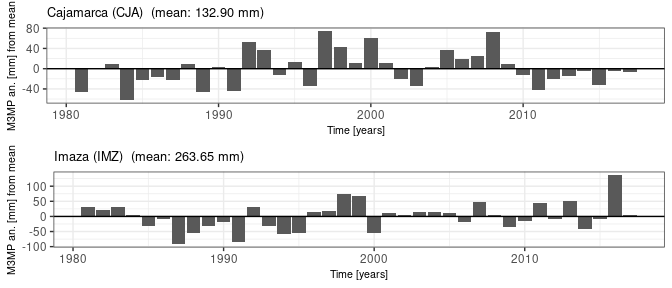r <-  prec_rank_mean_sens_slope_trend
#### Domain
mm <- range(r[],na.rm=TRUE)
buffer <- 1  #0.01
mm <- mm+c(-1,1)*buffer
minzero <- TRUE
if (minzero==TRUE) mm <- 0 ## Precipitation cannot be negative
step <- buffer ###10^(ceiling(log(mm)/log(10))-2)
domain=seq(from=mm,to=mm+step,by=step)

bins <- (length(domain))/2+1
color <- colorBin("YlGnBu", domain =domain,bin=bins)
values <- domain[domain<=max(r[])]

leaf_trend %>% addMarkers(lng=pts$lon,lat=pts$lat,label=sprintf("%10.2f mm/year at %s",r[pts$icell], pts$label))

The behavior of mean monthly precipitation within the rainy season is similar to the behavior of the total annual precipitation. Trends, where significantly exist, are not very high. However, mean monthly precipitation within the rainy season an indicator of rain water availability for agriculture during the growing season. In the following, both annual mean precipitation and mean monthly precipitation within the rainy season are rescaled and carried out homogenized with the reference to the conditional expected value of the last year of the time series, i.e. 2017, in order that the time series values are assumed to be independent and identically distributed:


years <- as.numeric(str_replace(names(prec_sum),"X",""))
years_max <- years[length(years)]
prec_sum_res <- prec_sum-stack(lapply(X=(years-years_max),FUN="*",prec_sum_sens_slope_trend))
prec_rank_mean_res <- prec_rank_mean-stack(lapply(X=(years-years_max),FUN="*",prec_rank_mean_sens_slope_trend))

Subsequently, package RasterList allows further analysis to assess the return periods of a specific scanario or a critical event (e.g. an extreme event). Through the L-Moments (Hosking and Wallis (1997)) a parametric probability distribution of precipitation is fitted for each raster cell. The function samlmu of lmom package (Hosking (2017)) is used to calculate the L-Moments of a generic time series. Precipitation variable sample is fitted with a Pearson Type III and a Gumbel Extreme Value probability distributions (Hosking (2017),Cordano (2022)), then the fit per each distribution is verified through a Kolgomorov-Smirnov statistical test (e.g. the ks.test function, Marsaglia, Tsang, and Wang (2003)). In the following chuck of code, this procedure is applied to the variable prec_rank_mean_res for one of the check points:

  library(lmomPi)
prec_m_ts <- prec_rank_mean_res[pts$icell] lmoments <- samlmu(prec_m_ts,ratios=TRUE) para <- pel_lmom(lmoments,distrib=c("pe3","gev")) para #>$pe3
#>          mu       sigma       gamma
#> 132.8999427  34.6265267   0.6304007
#> attr(,"probability_distrib")
#>  "pe3"
#>
#> $gev #> xi alpha k #> 118.2596808 30.4406342 0.1066909 #> attr(,"probability_distrib") #>  "gev" ks <- list() for (it in names(para)) { ks[[it]] <- ks.test(x=prec_m_ts,y="cdf",para=para[[it]]) } ks #>$pe3
#>
#>  Exact one-sample Kolmogorov-Smirnov test
#>
#> data:  prec_m_ts
#> D = 0.071272, p-value = 0.985
#> alternative hypothesis: two-sided
#>
#>
#> $gev #> #> Exact one-sample Kolmogorov-Smirnov test #> #> data: prec_m_ts #> D = 0.070062, p-value = 0.9875 #> alternative hypothesis: two-sided The procedure is therefore replicated at a raster scale (for each pixel of the raster) after defining and creating the following structures: == samlmom a RasterStack-class Object containing L-Moments for each pixel; == fitdist a RasterList-class object describing the probability distribution for each pixel with its parameters; == kstesting, a RasterList-class containing the output of the Kolgomorov-Smirnov Goodness-of-fit test for each pixel. These objects are built for each hypothetical probability distribution. samlmom <- stack(rasterList(prec_rank_mean_res,FUN=samlmu,ratios=TRUE)) ## This is a correction inas <- is.na(samlmom) samlmom[inas] <- 0 prec_rank_mean_res[inas] <- -9999 distrib <- c("pe3","gev") fitdist <- list() kstesting <- list() for (it in distrib) { fitdist[[it]] <- rasterList(samlmom,FUN=pel_lmom,distrib=it) kstesting[[it]] <- RasterListApply(x=rasterList(prec_rank_mean_res),y="cdf",para=fitdist[[it]],FUN=ks.test) } kstesting[["pe3"]]@list[[pts$icell]]
#>
#>  Exact one-sample Kolmogorov-Smirnov test
#>
#> data:  dots[[1L]][[435L]]
#> D = 0.071272, p-value = 0.985
#> alternative hypothesis: two-sided

Mapping p.value is useful to assess where the null hypothesis (e.g. the probability distrubution, in this case) can be accepted or not. Both selected probability distributions can be accepted for all raster cells.

  pval_ks <- list()
pval_ks[["pe3"]] <- raster(kstesting[["pe3"]],FUN=function(x){x$p.value}) plot(pval_ks[["pe3"]]>0.1)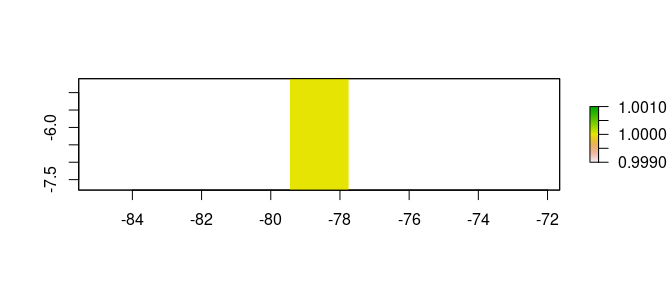pval_ks[["pe3"]] #> class : RasterLayer #> dimensions : 32, 17, 544 (nrow, ncol, ncell) #> resolution : 0.1, 0.1 (x, y) #> extent : -79.45, -77.75, -7.8, -4.6 (xmin, xmax, ymin, ymax) #> crs : +proj=longlat +datum=WGS84 +no_defs #> source : memory #> names : X1981 #> values : 0.2305134, 0.9999849 (min, max)  pval_ks[["gev"]] <- raster(kstesting[["gev"]],FUN=function(x){x$p.value})
plot(pval_ks[["gev"]]>0.1)pval_ks[["gev"]]
#> class      : RasterLayer
#> dimensions : 32, 17, 544  (nrow, ncol, ncell)
#> resolution : 0.1, 0.1  (x, y)
#> extent     : -79.45, -77.75, -7.8, -4.6  (xmin, xmax, ymin, ymax)
#> crs        : +proj=longlat +datum=WGS84 +no_defs
#> source     : memory
#> names      : X1981
#> values     : 0.3290517, 0.9999949  (min, max)

Return period is the expected time interval between two events where precipitation is lower than a certain threshold value. Return periods reveals the frequency at which specific events occurs. Since the object is to be analyze the meteorological drought (i.e. lack of rainfall), return periods are the inverse of quantiles associated to a precipitation lower that the mapped value. Under the hypothesis that the probability distribution is Pearson III type (i.e. pe3), return periods are calculated as follows:

return_periods <- c(5,10,20,50,100)
frq= 1/return_periods
names(frq) <- sprintf("T%d",return_periods)
percentiles <-  stack(fitdist[["pe3"]],FUN=function(p,frq) {
o <- qua(f=frq,para=p)
names(o) <- names(frq)

return(o)
},frq=frq)

Finally, here is a visualization of the value of mean monthly precipitation in the 3 most rainy months of the year that is not reached with a return period of 20 years:

r <-  percentiles[["T20"]]
rp <- r
r[r>1000] <- 1000
#### Domain
mm <- range(r[],na.rm=TRUE)
buffer <- 20  #0.01
mm <- mm+c(-1,1)*buffer
minzero <- TRUE
if (minzero==TRUE) mm <- 0 ## Precipitation cannot be negative
step <- buffer ###10^(ceiling(log(mm)/log(10))-2)
domain=seq(from=mm,to=mm+step,by=step)

bins <- (length(domain))/2+1
color <- colorBin("YlGnBu", domain =domain,bin=bins)
values <- domain[domain<=max(r[])]

leaf_trp %>% addMarkers(lng=pts$lon,lat=pts$lat,label=sprintf("%10.2f mm at %s",rp[pts$icell], pts$label))